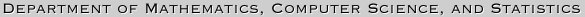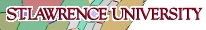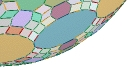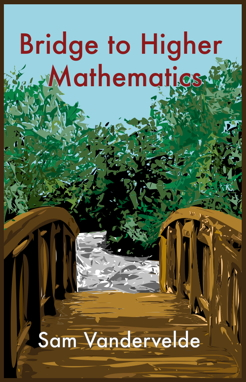What is this object? ...

Bridge To Higher Mathematics,
by Sam Vandervelde
..

 This textbook is designed to equip students who have completed a standard high school math curriculum with the tools and techniques that they will need to succeed in upper level math courses. Topics covered include logic and set theory, proof techniques, number theory, counting, induction, relations, functions, and cardinality. A significant portion of the book is available for download, in order to review the book or learn material. Click on the links below to obtain the desired sections of the text. Front matter - Table of Contents, etc. Chapter One - Logical Foundations Chapter Two - Set Theory Chapter Three - Proof Techniques Chapter Eight - Answers to all exercises Bridge to Higher Mathematics is available at www.lulu.com and also at amazon.com. The list price is \$32 at either site; the book itself is published through and printed by lulu.com.All known errors were corrected in May 2013, at which point small changes were also made to the wording in several places, one paragraph involving Fibonacci numbers was rewritten to implement a better technique, and two exercises changed to reflect this new approach.  However, in all other respects the current version of the second edition is identical to the original and can be used in its place without any difficulty.  As always, please do not hesitate to contact the author if you believe that you have found an error in the text.

The following excerpt is taken from the back cover of the book.

The text incorporates unique features such as Concept Checks and Mathematical Outings to actively engage the reader in the development of new ideas. The author, who writes questions for the USA Math Olympiad, has composed hundreds of thought-provoking problems to complement the lively exposition. Each chapter concludes with a reference section that concisely summarizes the definitions and proof strategies introduced in that chapter. Finally, answers to all the exercises and hints to every writing problem are included in the final chapter.

..

### ..Errata Page for Bridge to Higher Mathematics (current edition)

1. In an attempt to "improve" Problem 1.10 it was rendered impossible.  (Alas.)  The question should ask for the fourth and thirteenth student, not the third and fourteenth.

### ..Errata Page for Bridge to Higher Mathematics (for copies obtained prior to June 2013)

1. The abbreviation EOR for “exclusive or” used in Chapter One is not standard. The more commonly employed abbreviation is XOR.
2. The answer to Problem 1.41 should refer to eight people rather than ten, of course.
3. The answer to Problem 1.50a on page 208 should read "If 3^k+1 is a multiple of 4 then k is odd."  Furthermore, the answer to 1.50c is "If a is positive then 2^x=a has a solution."  How do these mistakes creep in so relentlessly, I wonder.
4. To avoid dealing with degenerate triangles, problem 1.71 would be better stated as "Explain why given any finite collection of three or more points in the plane, no three of which are collinear, there exists a triangle..."
5. The answer for Exercise 2.9b should read { 2^(n-1) + 1  |  n in N }, or perhaps more naturally as { 2^n + 1  |  n in Z, n≥0 }.  Alternatively, change the set appearing in the problem to be just {3, 5, 9, 17, 33, 65, ... }.
6. The answer for Exercise 2.10 should specify that m≥1, k≥0.
7. Exercise 2.41 should read:  If C={s, a, t, i, n} then how many sets D satisfy...  (The word `many' was omitted.)
8. Exercise 3.1h on page 57 is better stated as “... A is not a subset of B.”
9. The answer to Exercise 3.8a on page 213 should involve the cubes of m and n as opposed to their squares, while answer 3.8b should have x≤1.
10. The list of divisors of 24 in the answer to Exercise 4.2 on page 216 inadvertently leaves off 8 and -8.  (I used to know my divisors of 24...)
11. Alas, the assertion in Exercise 4.13 is true; just take a = 1.  The statement should read “distinct positive integers a,b>1.”
12. The answer to Exercise 4.58 on page 219 should read {-(k–1), -(k–2), ..., k–1, k}.  (It would also be fine to list the numbers from -k to k–1.)
13. On page 99 in Section 4.5 the Quick Query b) answer should state "0+0+1=1," not "0+1+1=1."  Ay, caramba!
14. The answer to Exercise 4.86 on page 220 makes no sense because we updated this problem but neglected to also update the answer.  The actual conjecture is that every odd number above 10 can be obtained in this manner except for 15.  (Note that the primes used must be distinct, so 15=5+2(5) doesn't work.)
15. There is a typo in the answer to Exercise 4.88 on page 220; the counterexample is 232+1.
16. Problem 5.13 on page 113 should have specified distinct nonzero digits.
17. Problem 5.63 on page 127 refers to a Mathematical Outing from Section 5.1, when in actuality it was from Section 5.3.  Oops.
18. The hint for Problem 6.35 on page 226 is inadequate since it only applies when r and s are positive rationals.  A better approach would be to suppose that r/s=a and s/r=b for natural numbers a and b.  Then multiply these equalities to get ab=1, which forces a=b=1, hence r=s.
19. The statement of Writing Problem 7.9 on page 174 should read “if and only if ”.  (The second  “if ” was accidentally omitted.)
20. The answer to Exercise 7.3 should read f(n)=3/4(n–1).  (The formula for g(n) is fine.)
21. The answer for Exercise 7.34 should refer to the point (3,0), rather than (3.0).  (Replace the decimal point with a comma.)
22. Sample Proof 7.1 on page 201 should use g(x) in the displayed equation rather than f(x).
23. The answer to Concept Check d) on page 195 inexplicably claims that ln 3 maps to 1/4.  In fact, ln 3 maps to 3/4, which has binary representation .110000..., leading to the correct answer of {1,2}.

...